# 目录

6.1 什么是数组

6.2 C语言二维数组

6.3 判断数组中是否包含某个元素

6.4 字符数组和字符串

6.5 排序问题

字符串数组

# 一、基础知识点

## 6.1什么是数组

1. #include
2. #include
3. int main()
4. {
5. int a1=20, a2=345, a3=700, a4=22;
6. int b1=56720, b2=9999, b3=20098, b4=2;
7. int c1=233, c2=205, c3=1, c4=6666;
8. int d1=34, d2=0, d3=23, d4=23006783;
9. printf("%-9d %-9d %-9d %-9d\n", a1, a2, a3, a4);
10. printf("%-9d %-9d %-9d %-9d\n", b1, b2, b3, b4);
11. printf("%-9d %-9d %-9d %-9d\n", c1, c2, c3, c4);
12. printf("%-9d %-9d %-9d %-9d\n", d1, d2, d3, d4);
13. system("pause");
14. return 0;
15. }

运行结果：

20        345       700       22
56720     9999      20098     2
233       205       1         6666
34        0         23        23006783  

为了减少变量的数量，让开发更有效率，使用数组（Array）为多个数据定义一个变量。

#include<stdio.h>
int main()
{
int i;
int a={20,345,700,22},
b={56720,9999,20098,2},
c={233,205,1,6666},
d={34,0,23,23006783};
for(i=0;i<4;i++)
{
printf("  %-9d",a[i]);
}
printf("\n");
for(i=0;i<4;i++)
{
printf("  %-9d",b[i]);
}
printf("\n");
for(i=0;i<4;i++)
{
printf("  %-9d",c[i]);
}
printf("\n");
for(i=0;i<4;i++)
{
printf("  %-9d",d[i]);
}
return 0;
}




#include <stdio.h>
int main()
{
int a = {20, 345, 700, 22};
int b = {56720, 9999, 20098, 2};
int c = {233, 205, 1, 6666};
int d = {34, 0, 23, 23006783};

printf("%-9d %-9d %-9d %-9d\n", a, a, a, a);
printf("%-9d %-9d %-9d %-9d\n", b, b, b, b);
printf("%-9d %-9d %-9d %-9d\n", c, c, c, c);
printf("%-9d %-9d %-9d %-9d\n", d, d, d, d);

return 0;
}

## 数组的概念和定义

arrayName 为数组名称，index 为下标。例如，a 表示第0个元素，a 表示第3个元素。

a=20;
a=345;
a=700;
a=22;

我们来总结一下数组的定义方式：

1. float m; //定义一个长度为 12 的浮点型数组
2. char ch; //定义一个长度为 9 的字符型数组
3. int a;//定义一个长度为4的整数型数组

对于数组的初始化需要注意以下几点：

1) 可以只给部分元素赋值。当{ }中值的个数少于元素个数时，只给前面部分元素赋值。例如：

int a={12, 19, 22 , 993, 344};

• 对于short、int、long，就是整数 0；
• 对于char，就是字符 '\0'；
• 对于float、double，就是小数 0.0。

int nums = {0};
char str = {0};
float scores = {0.0};    

2) 只能给元素逐个赋值，不能给数组整体赋值。例如给 10 个元素全部赋值为 1，只能写作：

int a = {1, 1, 1, 1, 1, 1, 1, 1, 1, 1};

而不能写作： int a = 1;

3) 如给全部元素赋值，那么在定义数组时可以不给出数组长度。例如：

int a[ ] = {1, 2, 3, 4, 5};

等价于

int a = {1, 2, 3, 4, 5};

（连续的内存为指针操作（通过指针来访问数组元素）和内存处理（整块内存的复制、写入等）提供了便利，这使得数组可以作为缓存（临时存储数据的一块内存）使用。）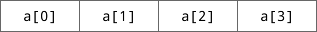#include <stdio.h>
int main()
{
int i,a;
//将1~10放入数组中
for(i=0; i<10; i++)
{
n[i] = (i+1);
}

//依次输出数组元素
for(i=0; i<10; i++)
{
printf("%d ", n[i]);
}

return 0;
}

1 2 3 4 5 6 7 8 9 10

更改上面的代码，让用户输入 10 个数字并放入数组中(从控制台读取用户输入)：

#include <stdio.h>
int main()
{
int i,a;

//从控制台读取用户输入
for(i=0; i<10; i++)             //数组 a 的最大下标是 9，也就是不能超过 10
{
scanf("%d", &a[i]);         //注意取地址符 &，不要遗忘哦
}

//依次输出数组元素
for(i=0; i<10; i++)
{
printf("%d ", a[i]);
}

return 0;
}

运行结果：

22 18 928 5 4 82 30 10 666 888↙

22 18 928 5 4 82 30 10 666 888

## 6.2C语言二维数组

int a;

a, a, a, a
a, a, a, a      如果想表示第 2 行第 1 列的元素，应该写作 a。
a, a, a, a

• 一种是按行排列， 即放完一行之后再放入第二行；在C语言中，二维数组是按行排列的。
• 另一种是按列排列， 即放完一列之后再放入第二列。

a, a, a, a------a行
a, a, a, a------a行
a, a, a, a------a行

## 二维数组的初始化（赋值）

1) 可以只对部分元素赋值，未赋值的元素自动取“零”值。例如：

int a = {{1}, {2}, {3}};     int a = {{0,1}, {0,0,2}, {3}};

1  0  0                                                                                   0 1 0
2  0  0                                                                                   0 0 2
3  0  0                                                                                   3 0 0

2) 如果对全部元素赋值，那么第一维的长度可以不给出。例如：

int a = {1, 2, 3, 4, 5, 6, 7, 8, 9};

int a[] = {1, 2, 3, 4, 5, 6, 7, 8, 9};

3) 二维数组可以看作是由一维数组嵌套而成的；如果一个数组的每个元素又是一个数组，那么它就是二维数组。当然，前提是各个元素的类型必须相同。根据这样的分析，一个二维数组也可以分解为多个一维数组，C语言允许这种分解。

a可以分解为a,a,a三个均有4个元素的一维数组，

a, a, a, a
a, a, a, a
a, a, a, a

## 6.3判断数组中是否包含某个元素

#include<stdio.h>
int main()
{
int i,n,thisindex=-1;
int a={1,10,6,296,177,23,0,100,34,999};
scanf("%d",&n);
for(i=0;i<10;i++)
{
if(a[i]==n)           //if括号中要用==，且后面用大括号{}
{
thisindex=i;      //把i赋值给thisindex
break;            //找到相等的数，打印下标，跳出循环
}
}
if(thisindex<0)
{
printf("%d isn't  in the array.\n",n);
}
else
{
printf("%d is in the array, it's index is %d.\n",n,thisindex);
}
}

100↙
100 is  in the array, it's index is 7.

28↙
28 isn't  in the array.

#include<stdio.h>
int main()
{
int i,n,thisindex=-1;
int a={1,10,6,296,177,23,0,100,34,999};
scanf("%d",&n);
for(i=0;i<10;i++)
{
if(a[i]==n)           //if括号中要用==，且后面用大括号{}
{
thisindex=i;      //把i赋值给thisindex
break;            //找到相等的数，打印下标，跳出循环
}
else if(a[i]>n) break;
}
if(thisindex<0)
{
printf("%d isn't  in the array.\n",n);
}
else
{
printf("%d is in the array, it's index is %d.\n",n,thisindex);
}
}

## 6.4C语言字符数组和字符串

1. char a; //一维字符数组
2. char b; //二维字符数组
3. char c={'c', ' ', 'p', 'r', 'o', 'g', 'r', 'a','m'}; // 给部分数组元素赋值
4. char d[ ]={'c', ' ', 'p', 'r', 'o', 'g', 'r', 'a', 'm' }; //对全体元素赋值时可以省去长度

C语言规定，可以将字符串直接赋值给字符数组，例如：

char str = {"wlacm.com"};

为了方便，也可以不指定数组长度，从而写作：

char str[] = {"wlacm.com"};

字符数组只有在定义时才能将整个字符串一次性地赋值给它，一旦定义完了，就只能一个字符一个字符地赋值了。

C语言在处理字符串时，会从前往后逐个扫描字符，一旦遇到'\0'就认为到达了字符串的末尾，就结束处理。'\0'至关重要，没有'\0'就意味着永远也到达不了字符串的结尾。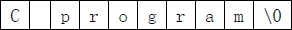" "包围的字符串会自动在末尾添加'\0'。例如，"C program"从表面看起来只包含了 9 个字符，其实不然，C语言会在最后隐式地添加一个'\0'，这个过程是在后台默默地进行的，所以我们感受不到。

char str = "abc123";

需要注意的是，逐个字符地给数组赋值并不会自动添加'\0'，例如：

char str[] = {'a', 'b', 'c'};

接下来没看懂的。。。

#include <stdio.h>
int main(){
char str;
char c;
int i;
for(c=65,i=0; c<=90; c++,i++){
str[i] = c;
}
str[i] = 0;             //此处为添加的代码，也可以写作 str[i] = '\0';
printf("%s\n", str);

return 0;
}

但是，这样的写法貌似有点业余，或者说不够简洁，更加专业的做法是将数组的所有元素都初始化为“零”值，这样才能够从根本上避免问题。再次修改上面的代码：

#include <stdio.h>
int main(){
char str = {0};  //将所有元素都初始化为 0，或者说 '\0'
char c;
int i;
for(c=65,i=0; c<=90; c++,i++){
str[i] = c;
}
printf("%s\n", str);

return 0;
}

#include <stdio.h>
#include <string.h>  //记得引入该头文件
int main(){
char str[] = "http://c.biancheng.net/c/";
long len = strlen(str);
printf("The lenth of the string is %ld.\n", len);

return 0;
}

## C语言字符串的输入和输出

• puts()：输出字符串并自动换行，该函数只能输出字符串。
• printf()：通过格式控制符 %s输出字符串，不能自动换行
#include <stdio.h>
int main(){
char str[] = "I.Love.You";
printf("%s\n", str);                            //通过字符串名字输出
printf("%s\n", "I.Love.You");       //直接输出
puts(str);                                      //通过字符串名字输出
puts("I.Love.You");                 //直接输出
return 0;
}

• scanf()：通过格式控制符%s输入字符串。
• gets()：直接输入字符串，并且只能输入字符串。

scanf() 和 gets() 是有区别的：

• scanf() 读取字符串时以空格为分隔，遇到空格就认为当前字符串结束了，所以***无法读取含有空格的字符串。***
• gets() 认为空格也是字符串的一部分，只有遇到回车键时才认为字符串输入结束，所以，不管输入了多少个空格，只要不按下回车键，对 gets() 来说就是一个完整的字符串。换句话说，***gets() 用来读取一整行字符串。***
#include <stdio.h>
int main()
{

char str1 = {0};
char str2 = {0};

//gets() 用法
printf("Input a string: ");
gets(str1);

//scanf() 用法
printf("Input a string: ");
scanf("%s", str2);    //注意这行scanf()

printf("str1: %s\n", str1);
printf("str2: %s\n", str2);

return 0;
}

## C语言字符串处理函数

C语言提供了丰富的字符串处理函数，可以对字符串进行输入、输出、合并、修改、比较、转换、复制、搜索等操作，使用这些现成的函数可以大大减轻我们的编程负担。

string.h是一个专门用来处理字符串的头文件

strcat 是 string catenate 的缩写，意思是把两个字符串拼接在一起，语法格式为：

strcat(arrayName1, arrayName2);

strcat() 将把 arrayName2 连接到 arrayName1 后面，并删除原来 arrayName1 最后的结束标志'\0'这意味着，arrayName1 必须足够长，要能够同时容纳 arrayName1 和 arrayName2，否则会越界（超出范围）。

strcat() 的返回值为 arrayName1 的地址。

#include<stdio.h>
#include<string.h>
int main()
{
char s1="AC ";
char s2;
gets(s2);
strcat(s1,s2);
puts(s1);
return 0;
}


is not the true happiness.

AC is not the true happiness.

strcpy 是 string copy 的缩写，意思是字符串复制，也即将字符串从一个地方复制到另外一个地方，语法格式为：

strcpy(arrayName1, arrayName2);

strcpy() 会把 arrayName2 中的字符串拷贝到 arrayName1 中，字符串结束标志'\0'也一同拷贝

strcpy() 要求 arrayName1 要有足够的长度，否则不能全部装入所拷贝的字符串。请看下面的例子：

#include <stdio.h>
#include <string.h>
int main(){
char str1 = "AC不是真正的快乐。";
char str2 = "AC is not the true happiness.";
strcpy(str1, str2);
printf("%s\n", str1);
return 0;
}

***字符串比较函数 strcmp()

strcmp 是 string compare 的缩写，意思是字符串比较，语法格式为：

strcmp(arrayName1, arrayName2);

#include <stdio.h>
#include <string.h>
int main(){
char a[] = "aBcDeF";
char b[] = "AbCdEf";
char c[] = "aacdef";
char d[] = "aBcDeF";
printf("a VS b: %d\n", strcmp(a, b));
printf("a VS c: %d\n", strcmp(a, c));
printf("a VS d: %d\n", strcmp(a, d));

return 0;
}

a VS b: 32
a VS c: -31
a VS d: 0

## 6.5排序问题

• 从数组头部开始，不断比较相邻的两个元素的大小，让较大的元素逐渐往后移动（交换两个元素的值），直到数组的末尾。经过第一轮的比较，就可以找到最大的元素，并将它移动到最后一个位置。
• 第一轮结束后，继续第二轮。仍然从数组头部开始比较，让较大的元素逐渐往后移动，直到数组的倒数第二个元素为止。经过第二轮的比较，就可以找到次大的元素，并将它放到倒数第二个位置。
• 以此类推，进行 n-1（n 为数组长度）轮“冒泡”后，就可以将所有的元素都排列好。

100

8 5

10 80 24

100

5 8

10 24 80

#include<stdio.h>
int main()
{
int i,j,t,n,a;

for(i=0;;i++)
{
if(scanf("%d",&a[i])!=1) break;
}
n=i;                    //读取数组时要令n有范围

for(i=0;i<n;i++)
{
for(j=i+1;j<n;j++)  //
{
if(a[i]>a[j])
{t=a[i];a[i]=a[j];a[j]=t;}
}
printf("%d ",a[i]);
}
}

a[i]             a[j]

（10               15     ）|      8           7          6          12          9

i=0,j=1,a=10与a=15两个相邻的数先进行比较，不用交换

a[i]         a[j]

10         |（  8            15  ）|       7          6          12          9

i=1,j=2,a=15与a=8进行比较，需要引入新变量t来达到交换的目的

10        8        7       6        12        9       15

8 7 6 10 9 12 15

7 6 8 9 10 12 15

6 7 8 9 10 12 15

#include<stdio.h>
int main()
{
int i,j,t,n,a;

for(i=0; ;i++)
{
if(scanf("%d",&a[i])!=1) break;
}
n=i;                    //读取数组时要令n有范围

for(i=1;i<n;i++)
{
for(j=0;j<n-i;j++)
{
if(a[j]>a[j+1])
{t=a[j];a[j]=a[j+1];a[j+1]=t;}
}
}

for(j=0;j<n;j++)
{
printf("%d ",a[j]);
}
}

# 二、典型例题

### 一维数组

#include<stdio.h>
int main()
{
int i,n=20,a;              //n=20,a只是定义时随意取值，不固定；
for(i=0;i<=n-1;i++)
{

if(scanf("%d",&a[i])!=1)   //if(scanf("%d",&n)==1)说明成功读入了n的值；
break;                     //用户端再输入完数组后输入字符显然不能成功读取时，
//便会结束循环,防止数组溢出；
}
n=i;                           //把此时结束循环时的i值赋给n，即要求的数据个数；
printf("%d",n);                //立刻输出而不能让n进入数组输出的循环结构中；
for(i=0;i<=n-1;i++)
{
printf(" %d",a[i]);        //注意输出数组时同样需要循环；
}
}

for(i=0;i<=n-1;i++)
{
if(scanf("%d",&a[i])!=1)    如果没有正确输入一个整型数给数组中的一个元素.
break;                                                   就会结束循环。
}

#include<stdio.h>
int main()
{
int i,n=20,a,k;
scanf("%d",&k);
for(i=0;i<=n-1;i++)
{
if(scanf("%d",&a[i])!=1) break;
}
n=i;                                       //这个条件不能少!!!
for(i=n-1;i>=0;i--)
{
printf(" %d",a[i]);
}
}


个人理解：n=i 这一行读取了输入的数组的数据长度，进而影响下面倒序输出数组时循环的条件。

成绩统计：低于平均值的人数与分数

#include<stdio.h>
int main()
{
int i,n=20,a,s=0;
float average;
for(i=0;i<=n-1;i++)
{
if(scanf("%d",&a[i])!=1) break;
s=s+a[i];
}
n=i;
average=(s*(1.0)/n);
int k=0,m;
for(i=0;i<=n-1;i++)
{
if(a[i]<average)
{
k=k+1;
}
}
printf("%d",k);
}


我的错误代码只算出了低于平均值的人数，分数没有搞定。

#include<stdio.h>
int main()
{
int i,n,a,s=0,k;
for(i=0;;i++)
{
if(scanf("%d",&a[i])!= 1) break;
s=s+a[i];
}
n=i;
k=0;
for(i=0;i<=n-1;i=i+1)
{
if(a[i]<1.0*s/n) k=k+1;
}
printf("%d",k);
for(i=0;i<=n-1;i=i+1)
{
if(a[i]<1.0*s/n)
printf(" %d",a[i]);
}

} 

有被自己蠢到😂

#include<stdio.h>
int main()
{
int i,n=20,a,s=0;
float average;
for(i=0;i<=n-1;i++)
{
if(scanf("%d",&a[i])!=1) break;
s=s+a[i];
}
n=i;
average=(s*(1.0)/n);
int k=0,m;
for(i=0;i<=n-1;i++)
{
if(a[i]<average)
{
k=k+1;
}
}
printf("%d",k);
for(i=0;i<=n-1;i++)
{
if(a[i]<average)
{
printf(" %d",a[i]);
}
}
}


成绩统计：最小值、最大值、平均值与均方差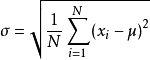，其中μ为数据的平均值

#include<stdio.h>
#include<math.h>
int main()
{
int i,n=20,a,s=0;
float u;
for(i=0;i<n;i++)
{
if(scanf("%d",&a[i])!=1) break;
s=s+a[i];
}
n=i;

u=s*(1.0)/n;
printf("%d %d %0.1f",a,a[n-1],u);
float o,m;
int p=0;
for(i=0;i<n;i++)
{
m=(a[i]-u)*(a[i]-u);
p=p+m;
}
o=sqrt((p*1.0)/n);
printf(" %0.2f",o);
}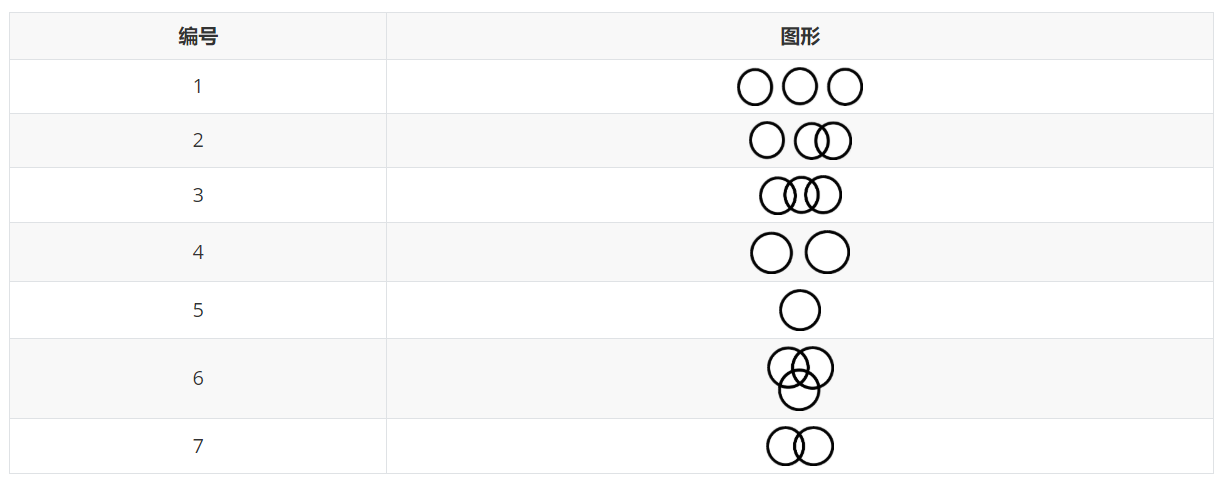输入：

PS:请注意π请使用3.14进行计算

对于每一组输入样例，按照样例格式输出，结果只保留整数部分，样例编号从1开始。

样例输入：

3
2 4 12.56
6.5 6 5 2.1
3 7 2

样例输出：

#Case1 : 25
#Case2 : 385
#Case3 : 54

### 二维数组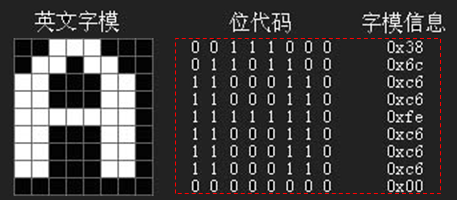输入：

输出：

8
0   0   1   1   1   0   0   0
0   1   1   0   1   1   0   0
1   1   0   0   0   1   1   0
1   1   0   0   0   1   1   0
1   1   1   1   1   1   1   0
1   1   0   0   0   1   1   0
1   1   0   0   0   1   1   0
0   0   0   0   0   0   0   0

样例输出：

ooo
oo  oo
oo      oo
oo      oo
ooooooo
oo      oo
oo      oo

又没复盘出来

#include<stdio.h>
int main()
{
int a,n;
scanf("%d\n",&n);

int i,j;
scanf("%d",a[i][j]);
for(i=0;i<n;i++)
{
for(j=0;j<n;j++)
{
if(a[i][j]==0) printf(" ");
if(a[i][j]==1) printf("o");
}
}
}

#include<stdio.h>
int main()
{
int i,j,n,a;
scanf("%d",&n);
for(i=0;i<=n-1;i=i+1)
{
for(j=0;j<=n-1;j=j+1)
{
scanf("%d",&a[i][j]);
}
}

for(i=0;i<=n-1;i=i+1)
{
for(j=0;j<=n-1;j=j+1)
{
if(a[i][j]==1) printf("o");
else printf(" ");
printf("\n");
}
}
}

矩阵转置

样例输入：

2 3
1 2 3
4 5 6

3 2
1 4
2 5
3 6

样例输出：

 1 4
2 5
3 6

1 2 3
4 5 6

#include<stdio.h>
int main()
{
int i,j,m,n,a;
scanf("%d %d",&m,&n);
for(i=0;i<=m-1;i=i+1)
{
for(j=0;j<=n-1;j=j+1)
{
scanf("%d",&a[i][j]);
}
}
for(j=0;j<=n-1;j=j+1)
{
for(i=0;i<=m-1;i=i+1)
{
printf(" %d",a[i][j]);
}
printf("\n");
}
}


#include<stdio.h>
int main()
{
int a,i,j,n;
scanf("%d",&n);
for(i=0;i<n;i++)
{
a=1;
a[i][i]=1;
for(j=0;j<n;j++)
{
if(i>=j) a[i][j]=a[i-1][j]+a[i-1][j-1];
else printf(" ");
}
}

for(i=0;i<n;i++)
{
for(j=0;j<n;j++)
{
printf("%d",a[i][j]);
}
printf("\n");
}
}



有错误      if(i>=j) a[i][j]=a[i-1][j]+a[i-1][j-1];   ？？？

#include<stdio.h>
int main()
{
int n,i,j,a;
scanf("%d",&n);
for(i=0;i<=n;i=i+1)
{
a[i]=1;
for(j=1;j<=i;j=j+1)
{
if(i==j) a[i][j]=1;
else a[i][j]=a[i-1][j]+a[i-1][j-1];
}
}
for(i=0;i<=n;i=i+1)
{
for(j=0;j<=i;j=j+1)
printf("%d ",a[i][j]);
printf("\n");
}
}


#include<stdio.h>
int main()
{
int i,j,n,a;
scanf("%d",&n);
for(i=0;i<n;i++)
{
for(j=0;j<=i;j++)
{
if(j==0||j==i) a[i][j]=1;
else a[i][j]=a[i-1][j]+a[i-1][j-1];
}
}

for(i=0;i<n;i++)
{
for(j=0;j<=i;j++)
{
printf("%d",a[i][j]);
}
printf("\n");
}
}


### 字符串数组

flow

wolf

正解

1.

#include<stdio.h>
#include<string.h>
int main()
{
char a,i,n;
gets(a);
n = strlen(a);
for(i=n-1;i>=0;i=i-1)
printf("%c",a[i]);
}

2.


#include<string.h>
#include<stdio.h>
int main()
{
char s;
int i,n,j;
gets(s);
for(i=0; ;i++)
{
if(s[i]=='\0')  break;
}
n=i;
for(j=n-1;j>=0;j--)
{
printf("%c",s[j]);
}
}



运行错误或答案错误非正解

#include<stdio.h>
#include<string.h>
int main()
{
char a;
int n,i,j,t;
gets(a);
n=strlen(a);
for(i=0,j=n-1;i<=j;i++,j--)
{
t=a[i];
a[i]=a[j];
a[j]=t;
}
for(i=0;i<n;i++)
{
printf("%c",a[i]);
}
}



#include<stdio.h>
#include<string.h>              //记得引入该头文件
int main()
{
char a,i,n;
gets(a);                   // gets()
n = strlen(a);
for(i=n-1;i>=0;i=i-1)
printf("%c",a[i]);         //     %c
}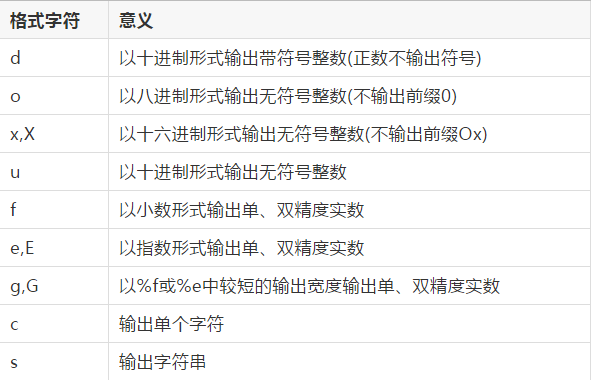【字符串】回文

yes 或 no 表示是否为回文

#include<stdio.h>
#include<string.h>
int  main(void)
{
int i,j;
int len;         //用于记录字符串长度
char S1;
char S2;

gets(S1);
len = strlen(S1);
for(i=len-1,j=0;i>=0;i--,j++)
{
S2[j] = S1[i];               //将S1逆序赋给S2
}
for(i=0,j=0;i<len;i++,j++)
{
if(S1[i]!=S2[i])                   //如果S1正序和逆序不同，则不是回文字符串
{
printf("no");
break;
}
else if(i==len-1)
{
printf("yes");
}
}
return 0;
}


答案错误25%

#include<stdio.h>
#include<string.h>
int main()
{
char s;
gets(s);
int i,j,n,k=0;
n=strlen(s);
for(i=0,j=n-1;i<=j;i++,j--)
{
if(s[i]!=s[j]) k=k+1; break;
}
if(k==0) printf("yes");
if(k==1) printf("no");
}


答案错误25%

#include<stdio.h>
#include<string.h>
int main()
{
char s;
gets(s);
int i,j,n,k=0;
n=strlen(s);
for(i=0,j=n-1;i<=j;i++,j--)
{
if(s[i]!=s[j]) k=k+1; break;
}
if(k) printf("no");   //啥意思
else printf("yes");
}


#include <stdio.h>
#include <string.h>
int main()
{
char str;
int i,n,k=0;
gets(str);
n = strlen(str);
for (i = 0; i <= n/2; i++ )
{
if (str[i] != str[n - 1 - i])
{
k=k+1;break;
}
}
if(k==0)  printf("yes");
if(k==1)  printf("no");
}

运行错误 50%

#include <stdio.h>
#include <string.h>
int main()
{
char str;
int i,n;
gets(str);
n = strlen(str);
for (i = 0; i <= n/2; i++ )
{
if (str[i] != str[n - 1 - i])
{
printf("no");
break;
}
else
if (i == n / 2)
printf("yes");
}
}

纠正英文语句中的大小写拼写错误

hello,How are you.

Hello,how are you.

#include<stdio.h>
#include<string.h>
int main()
{
char a;
gets(a);
int i,n,m;
n=strlen(a);
for(i=0;i<=n-1;i++)
{
if(a>=91) a=a-32;
if(a[i]==44)
{
if(a[i+1]<=91&&a[i+1]>=65) a[i+1]=a[i+1]+32;
}
printf("%c",a[i]);
}
}


#include
int main()
{
char s=44;
printf("%c",s);
}

,

# 总结

11-29
05-251189
05-25243
04-07
05-297279
11-202601
03-245万+
05-30835
12-111361
09-251658
02-01
02-284619
08-02
10-19874
05-293025
06-21635
09-01201
08-091万+
07-071万+

### “相关推荐”对你有帮助么？

•非常没帮助
•没帮助
•一般
•有帮助
•非常有帮助被折叠的  条评论 为什么被折叠?到【灌水乐园】发言¥2 ¥4 ¥6 ¥10 ¥20余额支付 (余额：-- )扫码支付获取中扫码支付点击重新获取扫码支付1.余额是钱包充值的虚拟货币，按照1:1的比例进行支付金额的抵扣。
2.余额无法直接购买下载，可以购买VIP、C币套餐、付费专栏及课程。余额充值